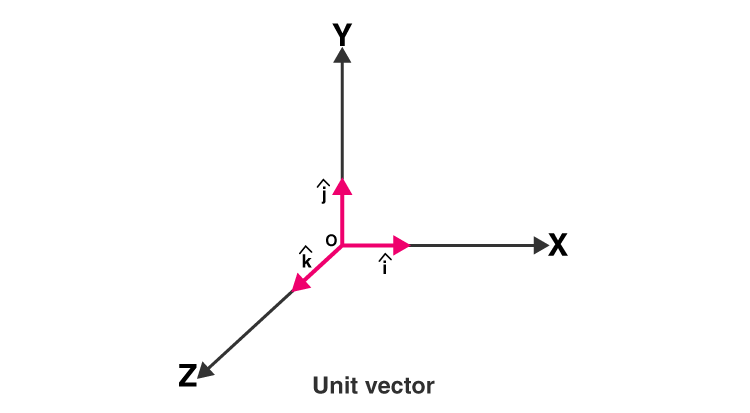# Unit Vector

A vector is a quantity that has both magnitude, as well as direction. A vector that has a magnitude of 1 is a unit vector. It is also known as Direction Vector.  Learn vectors in detail here.

For example, vector v = (1,3) is not a unit vector, because its magnitude is not equal to 1, i.e., |v| = √(12+32) ≠ 1. Any vector can become a unit vector by dividing it by the magnitude of the given vector.

## Unit Vector Symbol

Unit Vector is represented by the symbol ‘^’, which is called a cap or hat, such as: â. It is given by
$$\begin{array}{l}\hat{a}= \frac{a}{|a|}\end{array}$$

Where |a| is for norm or magnitude of vector a.

It can be calculated using a Unit vector formula or by using a calculator.Unit vectors are usually determined to form the base of a vector space. Every vector in the space can be expressed as a linear combination of unit vectors. The dot products of two unit vectors is a scalar quantity whereas the cross product of two arbitrary unit vectors results in third vector orthogonal to both of them.

 Related Articles Visualizing Unit Vectors Vectors Types of Vectors Vector Algebra for Class 12

What is the unit normal vector?

The normal vector is a vector which is perpendicular to the surface at a given point. It is also called “normal,” to a surface is a vector. When normals are estimated on closed surfaces, the normal pointing towards the interior of the surface and outward-pointing normal are usually discovered. The unit vector acquired by normalizing the normal vector is the unit normal vector, also known as the “unit normal.”Here, we divide a nonzero normal vector by its vector norm.

## Unit Vector Formula

As explained above vectors have both magnitude (Value) and a direction. They are shown with an arrow.

$$\begin{array}{l}i.e., \ \vec{a}\end{array}$$

And, â denotes a unit vector. If we want to change any vector in unit vector, divide it by the vector’s magnitude. Usually, xyz coordinates are used to write any vector.

It can be done in two ways:

1. $$\begin{array}{l}\vec{a} = (x, y, z)\ using\ the\ brackets\end{array}$$
2. $$\begin{array}{l}\vec{a}= x\hat{i} + y \hat{j} +z \hat{k}\end{array}$$

Formula for magnitude of a vector is:

 $$\begin{array}{l}\left | \vec{a} \right |=\sqrt{x^{2}+y^{2}+z^{2}}\end{array}$$ Unit Vector = Vector / Vector’s Magnitude

The above is a unit vector formula.

How to find the unit vector?

To find a unit vector with the same direction as a given vector, we divide the vector by its magnitude. For example, consider a vector v = (1, 4) which has a magnitude of |v|. If we divide each component of vector v by |v| we will get the unit vector uv which is in the same direction as v.

How to represent Vector in a bracket format?

$$\begin{array}{l}\hat{a} \hat{\equiv }\frac{a}{\left|a \right |} =\frac{(x,y,z)}{\sqrt{x^{2}+y^{2}+z^{2}}} =\frac{x}{\sqrt{x^{2}+y^{2}+z^{2}}},\frac{y}{\sqrt{x^{2}+y^{2}+z^{2}}},\frac{z}{\sqrt{x^{2}+y^{2}+z^{2}}}\end{array}$$

How to represent Vector in a unit vector component format?

$$\begin{array}{l}\hat{a}\hat{\equiv }\frac{a}{\left |a \right | } =\frac{x\hat{i}+ y\hat{j} +z \hat{k}}{\sqrt{x^{2}+y^{2}+z^{2}}} =(\frac{x}{\sqrt{x^{2}+y^{2}+z^{2}}}\hat{i},\frac{y}{\sqrt{x^{2}+y^{2}+z^{2}}}\hat{j},\frac{z}{\sqrt{x^{2}+y^{2}+z^{2}}}\hat{k})\end{array}$$

Where x, y, z are the value of the vector in the x, y, z axis, respectively and

$$\begin{array}{l}\hat{a} \ is\ a\ unit\ vector,\\ \vec{a} \ is\ a\ vector,\\\left | \vec{a} \right | \ is\ the\ magnitude\ of\ the\ vector\ \vec{a}, \\ \hat{i}, \hat{j}, \hat{k}\ are\ the\ directed\ unit\ vectors\ along\ the\ x,\ y,\ z\ axis.\end{array}$$

### Unit Vector Example

Here is an example based on the unit vector. Observe and follow each step and solve problems based on it.

Question 1:

$$\begin{array}{l}Find\ the\ unit\ vector\ \vec{p} \ for\ the\ given\ vector,\ 12\hat{i} – 3\hat{j} – 4 \hat{k}. \end{array}$$
Show it in both formats – Bracket and Unit vector component.

Solution:

Let’s find the magnitude of the given vector first,

$$\begin{array}{l}\left |p \right | = \sqrt{x^{2}+y^{2}+z^{2}} = \sqrt{144 + 9 + 16} = \sqrt{169}= 13\end{array}$$

Let’s use this magnitude to find the unit vector now:

$$\begin{array}{l}\hat{p} = \frac{p}{|p|} = \frac{x\hat{i}+y \hat{j} +z\hat{k}}{\sqrt{x^{2}+y^{2}+z^{2}}}\end{array}$$
$$\begin{array}{l}\hat{p}=\frac{12\hat{i}-3 \hat{j} – 4\hat{j}}{13}\end{array}$$
$$\begin{array}{l}\hat{p} = \frac{12}{13}\hat{i} -\frac{3}{13}\hat{j}-\frac{4}{13}\hat{k}\end{array}$$

The unit vector in Bracket form is:

$$\begin{array}{l}\hat{p} = (\frac{(12, -3, -4)}{13} = (\frac{(12)}{13}, -\frac{(3)}{13},\frac{(-4)}{13})\end{array}$$

### Unit Vector Problem

Question 2:

$$\begin{array}{l}Find\ the\ unit\ vector\ \vec{q} \ for\ the\ given\ vector,\ -2\hat{i} + 4\hat{j} – 4 \hat{k}.\end{array}$$
Show it in both the formats – Bracket and Unit vector component.

Solution:

Let’s find the magnitude of the given vector first,

$$\begin{array}{l}\left |q \right |=\sqrt{x^{2}+y^{2}+z^{2}}\end{array}$$
$$\begin{array}{l}\left |q \right | = \sqrt{-2^{2}+(4)^{2}+(-4)^{2}}\end{array}$$
$$\begin{array}{l}\left |q \right |=\sqrt{4 + 16 + 16}\end{array}$$
$$\begin{array}{l}\left |q \right |=\sqrt{36}\end{array}$$
$$\begin{array}{l}\left |q \right |= 6\end{array}$$

Let’s use this magnitude to find the unit vector now:

$$\begin{array}{l}\hat{q} = \frac{-2\hat{i} +4 \hat{j} – 4\hat{q}}{6}= \frac{-2}{6}\hat{i} + \frac{4}{6} \hat{j} -\frac{4}{6}\hat{k}\end{array}$$
$$\begin{array}{l}\hat{q} = \frac{-2}{6}\hat{i} + \frac{4}{6} \hat{j} -\frac{4}{6}\hat{k}\end{array}$$

The unit vector in Bracket form is:

$$\begin{array}{l}\hat{q} = \frac{(-2, 4, -4)}{6} = \frac{(-2)}{6}, \frac{(4)}{6}, \frac{(-4)}{6}= \frac{(-1)}{3}, \frac{(2)}{3}, \frac{(-2)}{3}\end{array}$$

To study more on Vectors and related Mathematical Topics, Visit BYJU’S.